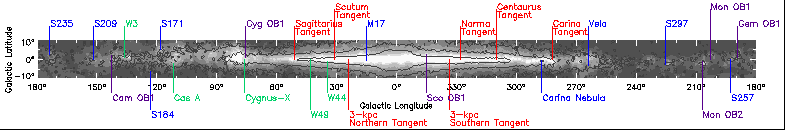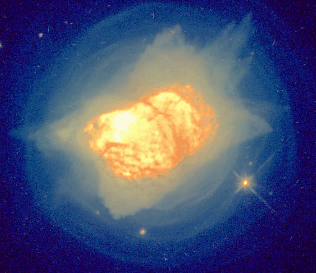# Introduction to Science in the Galactic Plane

## Lesson 5: Source Temperature### PURPOSE

This exercise will allow the instructor/teacher to present to his/her students a lesson based on the GPA Survey Angular Size exercise . Intensity values obtained in the previous exercise will be converted into temperature values for NGC 7027. This activity is usable for high school physical science, astronomy, and physics classes.

###Hubble Space Telescope Image of NGC 7027

### INTRODUCTION

The relationship between intensity and temperature in classical physics is based on the theory of BLACKBODY radiation. Using laws developed by Wien, Stefan, and Boltzmann, the theory describes how a heated body will radiate electromagnetic waves; visible light, radio waves, infrared, and ultraviolet. Ideal blackbodies emit electromagnetic radiation according to well defined laws that show the relationships among intensity, temperature, and frequency. This is true for stars. The source (NGC 7027) examined in the previous exercise may be treated as an ideal blackbody even though NGC 7027 is a planetary nebula. You will investigate this relationship during this exercise.

### THE PROJECT

In this project, you will use the previously found intensity values (in Jy) and the angular size (in arcsec) to calculate the temperature of NGC 7027. Once this calculation has been performed, compare your calculated temperature against an accepted value.

### ANALYSIS TO BE PERFORMED BY STUDENTS

(1) From a previous exercise, record the source intensity in Jy.
(2) From a previous exercise, record the angular size in arcsec.
(3) Apply a correction factor based on the beam size of the antenna system used to obtain the radio image.
(4) Calculate the temperature of NGC 7027 from the following formulae:

 (a) Equation a: Uncorrected temperature/beam = Source Flux * 0.392 K/beam. (b) Equation b: Correction factor = (beam size)^2/(angular size)^2 (c) Equation c: Object Temperature = (correction factor)*(temperature/beam).

Note: the antenna beam size is 670 arcsec at 8.35 GHz; 480 arcsec at 14.35 GHz.

The expanding shell of gas surrounding NGC 7027, called the Stellar Wind Region has an approximate temperature of 54 K at its base and 10 K at the outer boundary.* How does your temperature calculation compare with this range of values?

* The Astrophysical Journal, 532:994-1005, 2000, April 1.

Galactic Plane Science
2000-06-27

rwelsh@nrao.edu, glangsto@nrao.edu